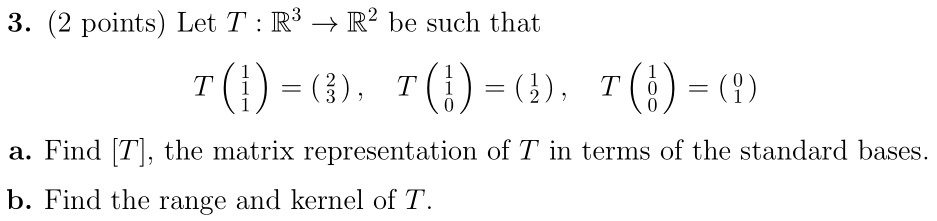Home / Answered Questions / Other / 3-2-points-let-t-r3-r2-be-such-that-t-1-3-7-7-a-find-t-the-matrix-representation-of-t-in-terms-of-th-aw800

# (Solved): 3. (2 Points) Let T : R3 â†’ R2 Be Such That T(1) = (3), 7(!) = (), 7(!) = (?) A. Find (T), The Matr...3. (2 points) Let T : R3 â†’ R2 be such that T(1) = (3), 7(!) = (), 7(!) = (?) a. Find (T), the matrix representation of T in terms of the standard bases. b. Find the range and kernel of T.

We have an Answer from Expert